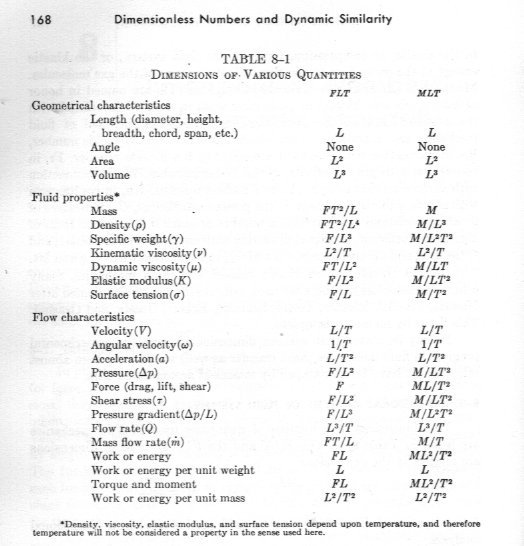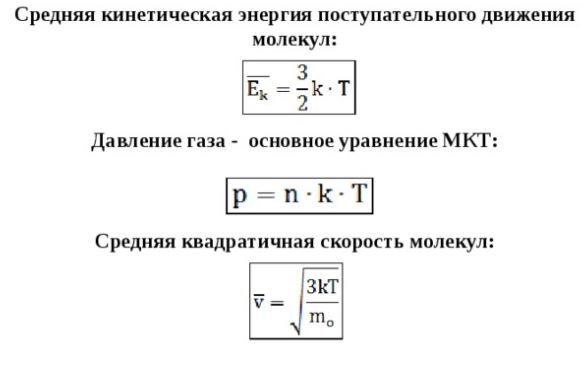# Доказательство гипотезы Больших Чисел Дирака. Proof of the Hypothesis of Large Dirac Numbers.

## Recommended Posts

1 hour ago, Aeromash said:

F=c4G

Force  in the SI system.

What?

1 hour ago, Aeromash said:

φ=v2=Gmr

The square of speed is the gravitational potential.

A car moving on the surface of the earth can have a wide range of speeds without changing its gravitational potential.

If you square GM/r you get G2M2/rwhich is not the force (GmM/r2)

##### Share on other sites

40 minutes ago, studiot said:

L-12 does not equal L-6L-5

Thanks! My mistake!

$L^{-12}=L^{-5}L^{-7}=F=qE$

$L^{-5}$

charge.

$L^{-7}$

electric field strength.

$L^{-6}$

Рotential.

Edited by Aeromash
##### Share on other sites

31 minutes ago, swansont said:
1 hour ago, Aeromash said:

φ=v2=Gmr

The square of speed is the gravitational potential.

The square of the velocity is not the gravitational potential, the gravitational potential is the square of the escape velocity.

##### Share on other sites

45 minutes ago, swansont said:

A car moving on the surface of the earth can have a wide range of speeds without changing its gravitational potential.

You are talking about the speed of the car. And not about the square of the speed or the gravitational potential of the car. You are confusing physical quantities.

##### Share on other sites

2 minutes ago, Aeromash said:

You are talking about the speed of the car. And not about the square of the speed or the gravitational potential of the car. You are confusing physical quantities.

You are confusing this. The gravitational potential of the Earth, not the car. If the car moves so fast that the square of its speed becomes equal to the gravitational potential of the Earth not on its surface, then the car will fly into the Solar System.

##### Share on other sites

49 minutes ago, swansont said:

If you square GM/r you get G2M2/rwhich is not the force (GmM/r2)

$\frac{\varphi^{2}}{G} =\frac{G^{2}M^{2}}{Gr^{2}}=F$

G is the constant of the transition from the LT system to SI.

##### Share on other sites

1 minute ago, Aeromash said:

φ2G=G2M2Gr2=F

G is the constant of the transition from the LT system to SI.

The force is a vector, not a scalar

##### Share on other sites

46 minutes ago, Aeromash said:

You are talking about the speed of the car. And not about the square of the speed or the gravitational potential of the car. You are confusing physical quantities.

If the speed of the car is variable then so is the square of the speed. I thought that would be self-evident. I did mention the gravitational potential; it is constant on the surface of the sphere of the earth.

If the square of the speed is variable, then it cannot be equal to a constant.

42 minutes ago, Aeromash said:

φ2G=G2M2Gr2=F

G is the constant of the transition from the LT system to SI.

Sorry, G is taken already, and this is a new fudge factor you have added because your original claim is wrong.

It's still not the force, because the gravitational potential does not include the mass of the object, and the force does. Squaring the potential will not make the mass of the object magically appear.

Using unit analysis gives you proportionalities, at best. Not equalities.

1 hour ago, SergUpstart said:

The square of the velocity is not the gravitational potential, the gravitational potential is the square of the escape velocity.

No, it's not. There's a pesky factor of 2 that need to be included. It has no units, so unit analysis and manipulation would not reveal it.

##### Share on other sites

14 hours ago, swansont said:

Using unit analysis gives you proportionalities, at best. Not equalities.

Yes! Using unit analysis gives you proportionalities. But using unit analysis gives me equalities in L and LT systems. For the transition to the SI system need the gravitational constant G or, in electrodynamics, epsilon . Newton knew nothing about the constant G. Mass in his era had dimensions:

$\frac{m^{3}}{s^{2}}$

In general, this is terrible, but the SI system is a mixture of the LMT, LT and L systems. Therefore, thinking that we are working in SI, in fact, we use the LT or L system. When we work in SI, then coefficients of the G or epsilon type appear in the formulas right away. When you calculate the volume in SI by multiplying the area by the height, you are actually working in the L system. If you added mass to the LT system, that is, switched to the LMT system, then the constant G immediately appears in the formulas. The larger the dimension of the system, the more different coefficients(constants) you need! This is what we observe in modern physics.

System L does not need coefficients! This is its beauty.

16 hours ago, SergUpstart said:

The force is a vector, not a scalar

Vector if the radius is a vector.

$\vec{r}$

Edited by Aeromash
##### Share on other sites

17 hours ago, swansont said:

A car moving on the surface of the earth can have a wide range of speeds without changing its gravitational potential.

A car moves at different speeds relative to the Earth's surface, but its speed does not exceed the square root of the gravitational potential at the Earth's surface. In this matter, it is a gravitationally bound object, which is one with the Earth. Unlike Tesla's car, which is part of the solar system.

##### Share on other sites

4 hours ago, Aeromash said:

Yes! Using unit analysis gives you proportionalities. But using unit analysis gives me equalities in L and LT systems. For the transition to the SI system need the gravitational constant G or, in electrodynamics, epsilon . Newton knew nothing about the constant G. Mass in his era had dimensions:

m3s2

In general, this is terrible, but the SI system is a mixture of the LMT, LT and L systems. Therefore, thinking that we are working in SI, in fact, we use the LT or L system. When we work in SI, then coefficients of the G or epsilon type appear in the formulas right away. When you calculate the volume in SI by multiplying the area by the height, you are actually working in the L system. If you added mass to the LT system, that is, switched to the LMT system, then the constant G immediately appears in the formulas. The larger the dimension of the system, the more different coefficients(constants) you need! This is what we observe in modern physics.

System L does not need coefficients! This is its beauty.

70 posts, some of them have involved a lot of work and I still don't understand the basis of what you are saying about dimensions. Most of what you have posted assumes the reader understands and agrees with your basic statement about dimensions.

I don't understand or agree.

You mention unit analysis and also Tesla.

There is a form of analysis in electrical power engineering called 'per unit' analysis.

I don't know if you are trying to emulate this.

The problem with 'unit analysis' is that the dimensions are hidden in the 'units' employed.

They haven't really gone away.

So can I respectfully suggest you put some of this effort into developing (proving) your underlying claim once and for all?

Perhaps showing one simple example developed from start to fininsh, but involving as many as possible of the seven basic quantities in the LMTAθIN system.

For your information here are two free websites where you build mathematical formulae and copy/paste into ScienceForums.

##### Share on other sites

3 hours ago, Aeromash said:

A car moves at different speeds relative to the Earth's surface, but its speed does not exceed the square root of the gravitational potential at the Earth's surface.

That was not your claim.  You said "The square of speed is the gravitational potential." with no caveats.

##### Share on other sites

Since I asked for a clear example it is only fair that I give you one showing what i mean.

On a per unit basis, the molecular weight of water is 18.

Superficially, that apppears to be a pure number.

But that is because the the real dimensions are hidden in the per unit, which is a gramme-molecule.

So a complete statement is the molecular weight of water is 18 grammes per gramme-molecule.

The interesting part of this story is that the full dimensional statement is MN, although the N is usually 'understood' and not stated explicitly.

Edited by studiot
##### Share on other sites

4 hours ago, studiot said:

Perhaps showing one simple example developed from start to fininsh, but involving as many as possible of the seven basic quantities in the LMTAθIN system.

A good question takes a long time to answer. I am preparing a general table of the transition of the one-dimensional laws of the system L to the SI system.

##### Share on other sites

1 hour ago, Aeromash said:

A good question takes a long time to answer. I am preparing a general table of the transition of the one-dimensional laws of the system L to the SI system.

If I can understand and agree with it I can then follow all your other long posts that have taken so much effort.

As a matter of interest the MLT system is not unique, nor is it specifically SI, although yes Système International d'Unités has adopted it.

But there are also other systems in use for instance one with mass replaced by force - the FLT system.

The FLT system finds favour in Fluid Mechanics as it make this system easier (at least for Fluid Mechanics) as can be seen from this small table. (after Olson :  Engineering Fluid Mechanics)Note at the bottom of the table the comment about temperature.

I did ask and you have not said how your L system handles temperature.

Edited by studiot
spelling
##### Share on other sites

17 hours ago, studiot said:

Note at the bottom of the table the comment about temperature.

I did ask and you have not said how your L system handles temperature.

$E \sim T^{\circ}$

Energy is proportional to temperature. Which means that the temperature has the same degree as the energy in the system  L.

$L^{-11}=T^{\circ}$##### Share on other sites

50 minutes ago, Aeromash said:

ET

Energy is proportional to temperature. Which means that the temperature has the same degree as the energy in the system  L.

L11=T

Really ?

What is n ?

That leaves me still waiting for some unit free constant that couples length to any one of the other independent physical dimensions I have mentioned.

Do you know what they are?

If not are you not curious about my symbols ?

I certainly don't agree that all forms of 'Energy' in general are proportional to temperature.

Edited by studiot
##### Share on other sites

35 minutes ago, studiot said:

What is n ?

##### Share on other sites

27 minutes ago, Aeromash said:

I don't think that answers the question

## Create an account

Register a new account